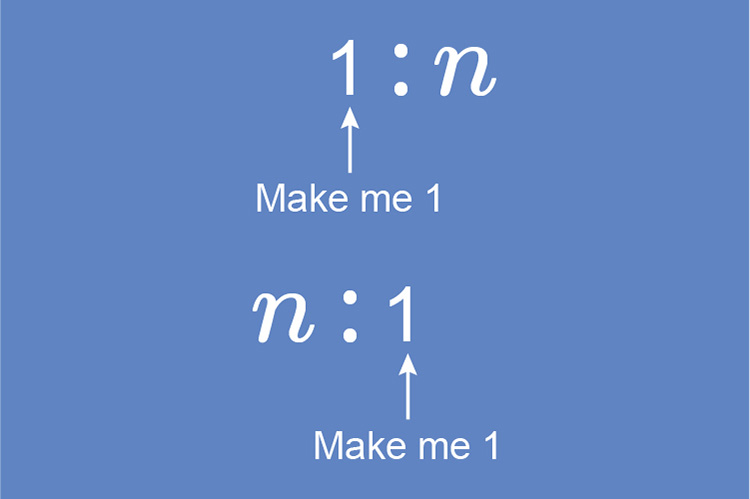# Ratios in the form 1:n or n:1

In order that a ratio is written in the form 1:n we must make the left hand side equal to one.

In order that a ratio is written in the form n:1 we must make the right hand side equal to one.NOTE:

n can be expressed as a decimal or a fraction

Example 1

Express 6:10 in the form 1:n

So the ratio is

6:10

and we need to work out

1:n

(see our section on percentages for full explanation)

6:10

1:n

6/1=10/n

Rearranging the formula

n=(10times1)/6

n=10/6

simplifying the fraction

n=5/3

So the ratio in the form 1:n is

1:5/3

Example 2

Express 6:10 in the form n:1

So the ratio is

6:10

and we need to work out

n:1

(see our section on percentages for full explanation)

6:10

n:1

6/n=10/1

Rearranging the formula

(6times1)/10=n  or

n=(6times1)/10

n=6/10

simplifying the fraction

n=3/5

So the ratio in the form n:1 is

3/5:1

Example 3

The ratio of girls to boys in a school is:

800 boys to 1200 girls

What is the ratio in the form n:1?

So the ratio is

800:1200

and we need to work out

n:1

(see our section on percentages for full explanation)

800:1200

n:1

800/n=1200/1

Rearranging the formula

(800times1)/1200=n  or

n=(800times1)/1200

n=800/1200

simplifying the fraction

n=(8cancel00)/(12cancel00)

n=8/12

simplify the fraction further

n=4/6

simplify the fraction further

n=2/3

This will not simplify any further so the answer is

2/3:1

Example 4

A chief is cooking a meal for many and she knows that she has to buy 3 carrots to 8 parsnips. She also knows that the ratio of parsnips to broccoli is 6 parsnips to 1 broccoli. Write out the ratio in the form A:B:C

The ratio is     3 carrots :8 parsnips and

6 parsnips :1 broccoli

Line these up we would have

3 carrots :8 parsnips and 6 parsnips :1 broccoli

We need to make the second ratio have 8 parsnips i.e. the same as the first ratio.

Therefore

6 parsnips :1

and we need to work out

8 parsnips :n

(see our section on percentages for full explanation)

6:1

8:n

6/8=1/n

rearranging the formula

n=(1times8)/6

n=8/6

Simplify the fraction

n=4/3

So the new ratio is

8 parsnips :4/3 broccoli

So the full ratio is

3 carrots :8 parsnips and 8 parsnips :4/3 broccoli

Which is the same as

3 carrots :8 parsnips :4/3 broccoli